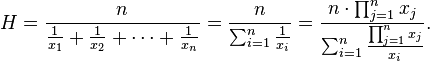## Harmonic Mean Calculator

 Enter Inputs 5,20,40,80,100 Enter the Numbers with Comma separated(,)
 Result: No.of Inputs Harmonic Mean

The Harmonic Mean Calculator to calculate Harmonic Mean from the number of observations, divided by the sum of reciprocals of the observations.

### Harmonic Mean FormulaWhere H is harmonic mean, x1, x2, x3, ...xn > 0.

For example, data set {5,20,40,80,100}, then Harmonic Mean = 16.8067.

Thinkcalculator.com provides you helpful and handy calculator resources.